U-statistic

A sum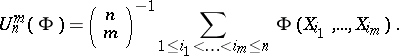Hoeffding's form for-statistics is [a1]: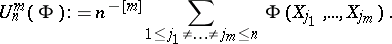The kernel of a-statistic,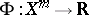, is a symmetric real-valued function ofvariables. The random variables(cf. also Random variable) are independent identically distributed with common distribution functionon a measurable space,. The number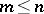is called the degree of the-statistic. The number of terms in the sum is equal to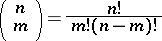in the first sum and to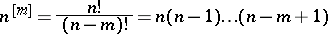in the second sum. Also,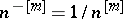.

Various statistics can be represented as-statistics or can be approximated by-statistics with a suitable choice of the kernel. For example, the sampling variance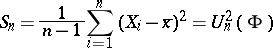can be obtained using the kernel. Here,is the mean value of the sample. The von Mises functional, given by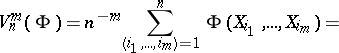where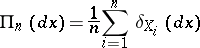is the empirical distribution, can be represented by a linear combination of-statistics [a2]. For the primitive kernel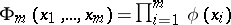, the-statistic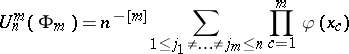is a symmetric polynomial statistic of the random variables,.

The starting point of the analysis of-statistics is the Hoeffding decomposition of-statistics, [a1]: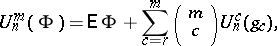where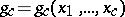,, are completely degenerate kernels: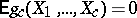. The integer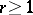is called the rank of the-statistic. Here, by definition,is the mean value of the kernel and, also,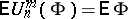. Therefore, an-statistic is an unbiased estimator of the functional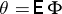.

The theory of-statistics, founded by W. Hoeffding in the seminal work [a1], published in 1948, was developed under the impact of the theory of sums of independent random variables. The law of large numbers, the central limit theorem, the law of the iterated logarithm, etc. were investigated in various works (see the references in [a3]). The asymptotic behaviour of-statistics can be reduced to the analysis of sums of independent identically distributed random variables. For a non-degenerate kernelwith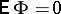and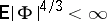there is weak convergence (as; cf. also Convergence, types of)):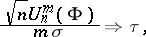whereis a random variable with standard Gaussian distribution with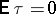and. Here,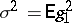.

For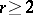the limit distribution of-statistics depends essentially on the kernel. For a primitive completely degenerate kernel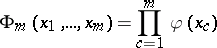withand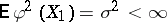, there is weak convergence (as):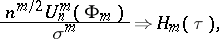whereis the Hermite polynomial of degree[a7] (cf. also Hermite polynomials).-statistics with completely degenerate kernel,and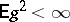, converge weakly (as) to the Itô–Wiener stochastic integral [a3], [a5]: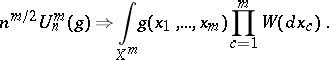-statistics can also be represented by the stochastic integral with respect to the permanent random measure, as follows, [a3],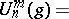where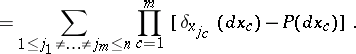The asymptotic analysis of-statistics is based on the martingale structure of-statistics and involves functional limit theorems, rate of convergence, almost sure convergence, asymptotic expansions, and probability of large deviations.

The contemporary development of the theory of-statistics contains various generalizations:-statistics with kernel taking values in a Hilbert or Banach space [a8], multi-sampling-statistics, bootstrap and truncated-statistics, weighted-statistics, etc.-statistics with kernel depending onare used in non-parametric density and regression estimation [a2], [a3], [a4], [a5], [a6].